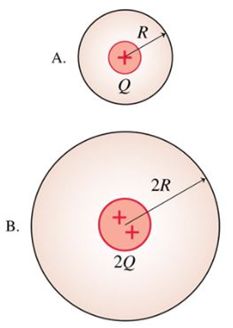# Problem: Which spherical Gaussian surface has the larger electric flux?a. Surface Ab. Surface Bc. They have the same flux

###### FREE Expert Solution

Electric flux is expressed as:

$\overline{){{\mathbf{\varphi }}}_{{\mathbf{E}}}{\mathbf{=}}\frac{{\mathbf{q}}_{\mathbf{e}\mathbf{n}\mathbf{c}\mathbf{l}\mathbf{o}\mathbf{s}\mathbf{e}\mathbf{d}}}{{\mathbf{\epsilon }}_{\mathbf{0}}}}$###### Problem Details

Which spherical Gaussian surface has the larger electric flux?a. Surface A
b. Surface B
c. They have the same flux